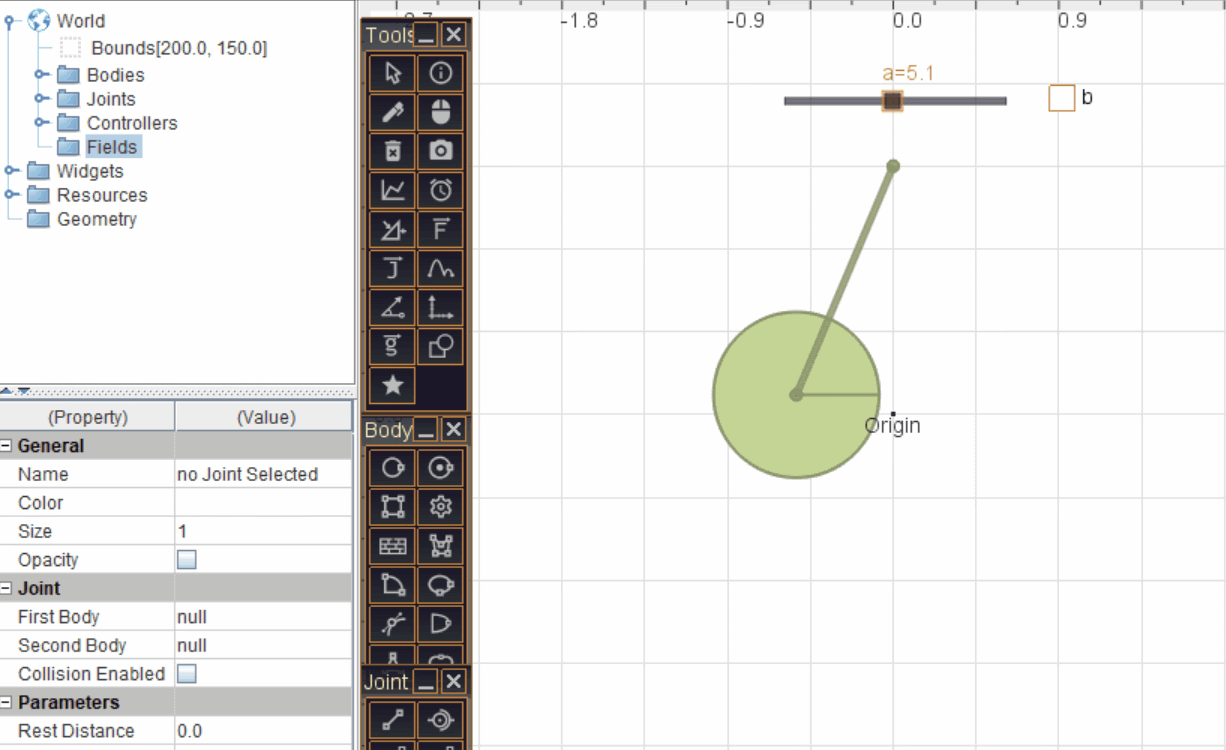# Field Forces

SimPhy supports setting up of various fields. Mentioned below are some of them-

Gravity: The default gravity is 9.8 m/s2 pointing in the negative y direction. You can set the Gravity Scale or Gravity factor (with default value 1) of individual objects to experience different gravity and behave differently in the Property Panel.

Electrostatic field: Electrostatic field (in N/C) added to the world, applies force on an object if and only if it has non zero charge and it is inside the bounds of the field.

The electrostatic force on an object is calculated as Force = charge x electrostatic field

Magnetic field: Magnetic field (in Wb/m2 perpendicular to the plane) added to the world, apply force on an object if and only if it has non zero charge and it is moving.

The magnetic lorentz force on an object is calculated as Force = charge x speed x magnetic field

and is perpendicular to the velocity

Buoyancy and drag: Buoyancy can be added to a region representing hypothetical liquid in that region. The density and viscosity of liquid can be changed while adding/editing field.

The buoyancy force on an object is calculated as

Force = [area submerged] X [density of liquid] X [gravity] -[viscosity]*[speed]

Additional correction for lift is also taken into account based on the geometry of the object.

Air Drag: Air drag can be simulated using Buoyancy field with small values of density (e.g. 0.001) and small value of viscosity (e.g 0.001).

You can easily add fields without any coding as shown in the GIF or simply by right clicking in the working area and then select world settings then choose desired Field.Note:

• Electric and magnetic forces will be experienced by bodies having non zero charge.
• For how to add change to body, have a look at property panel
• Fields like buoyancy have some default hard coded settings for drag coefficient etc.
• All Fields are measured in the SI System of units.
0 replies